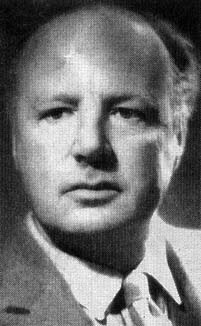A# Champernowne's number

Champernowne's number is the first known normal number. It was invented (or discovered) in 1933 by the English mathematician David G. Champernowne (1912–2000) and consists of a decimal fraction in which the decimal integers are written down in increasing order: 0.12345678910111213... Champernowne's number has been proven to be a normal number in base 10 and also to be an irrational number. However, although it is normal, so that its digits appear with equal frequency, the sequence of its digits are not unpredictable. An example of a number whose sequence of digits is unpredictable is Chaitin's constant.David Champernowne